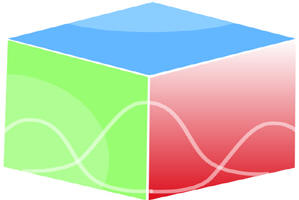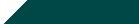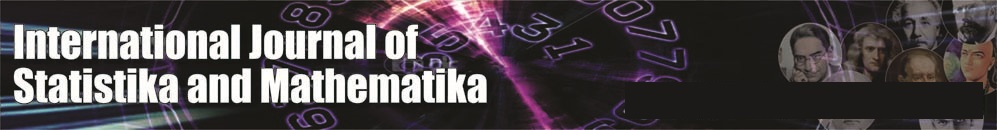``Home| Journals | Statistical Calculator | About Us | Contact UsUntitled Document

International Journal of Statistika and Mathematika, ISSN: 2277- 2790 E-ISSN: 2249-8605

Volume 3, Issue 1, 2012 pp 16-26

Research Article

Common Fixed Point Theorems for Sequence of Mappings in M - Fuzzy Metric Space

T. Veerapandi1, G. Uthaya Sankar2 and A. Subramanian3

1Associate Professor of Mathematics, P.M.T. College, Melaneelithanallur � 627953 INDIA

2Department of Mathematics, Mano College (An Institution of M.S. University, Tirunelveli), Sankarankovil � 627756 INDIA

3Department of Mathematics, The M.D.T. Hindu College, Tirunelveli � 627010 INDIA

        Dhage .B.C, Generalised metric spaces and mappings with fixed point, Bull. Calcutta Math. Soc., 84(4), (1992), 329-336.

        George .A and Veeramani .P, On some results in fuzzy metric space, Fuzzy Sets and Systems, 64 (1994), 395-399.

        Grabiec .M, Fixed points in fuzzy metric spaces, Fuzzy Sets and Systems, 27 (1988), 385-389.

        Gregori V and Sapena A, On fixed point theorem in fuzzy metric spaces, Fuzzy Sets and Systems, 125 (2002), 245-252.

        Klement .E.P, Mesiar .R and Pap .E, Triangular Norms, Kluwer Academic Publishers.

        Kramosil .I and Michalek .J, Fuzzy metric and statistical metric spaces, Kybernetica, 11 (1975), 326-334.

        Mihet D, A Banach contraction theorem in fuzzy metric spaces, Fuzzy Sets and Systems, 144 (2004), 431-439.

        Naidu .S.V.R, Rao .K.P.R and Srinivasa .R.N, On the topology of D-metric spaces and the generation of D-metric spaces from metric space, Internet. J. Math. Math. Sci., 51 (2004), 2719-2740.

        Naidu .S.V.R, Rao .K.P.R and Srinivasa .R.N, On the convergent sequences and fixed point theorems in D-metric spaces, Internet. J. Math. Math. Sci., 12 (2005), 1969-1988.

      Park .J.H, Park .J.S and Kwun .Y.C, Fixed point in M � fuzzy metric spaces, Optimization and Decision Making, 7 (2008), No.4, 305-315.

      Schweizer .B and Sklar .A, Statistical metric spaces, Pacific. J. Math., 10 (1960), 314-334.

      Sedghi .S and Shobe .N, A Common fixed point theorems in two M � fuzzy metric spaces, Commun. Korean Math. Soc. 22(2007), No.4, 513-526.

      Sedghi .S and Shobe .N, Fixed point theorem in M � fuzzy metric spaces with property (E), Advances in Fuzzy Mathematics, Vol.1, No.1 (2006), 55-65.

      Veerapandi .T, Jeyaraman .M and Paul Raj Josph .J, Some fixed point and coincident point theorem in generalized M � fuzzy metric space, Int. Journal of Math. Analysis, Vol.3, (2009), 627-635.

      Veeapandi .T, Uthaya Sankar .G and Subramanian .A, Some Common fixed point theorems in M � fuzzy metric space, Int. Journal of Mathematical Archive-3(3), (2012), 1005-1016.

      Zadeh .L. A, Fuzzy sets, Information and Control, 8 (1965), 338-353.